:::

# 多組常態分佈資料之差異檢定與事後比較：R的ANOVA與Welch's anova / Parametric Tests for Comparing Many Normal Distribution Groups: ANOVA and Welch's anova in R 1/28/2018 Programming/R , Statistics 8 Comments Edit Copy Download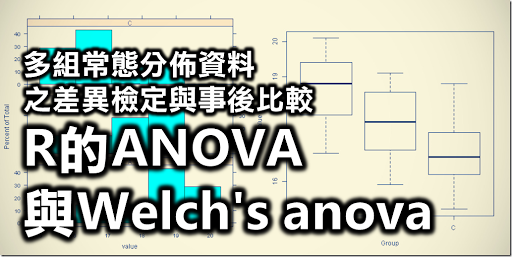#### R腳本與操作環境：RStudio / R script & Environment: RStudio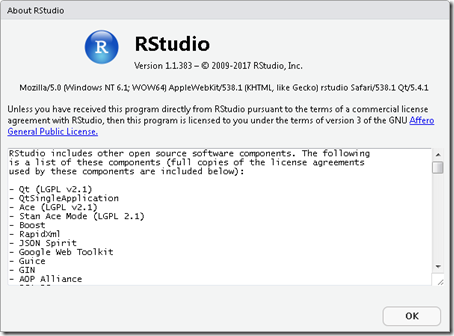R腳本必須要在R環境中執行，我推薦安裝RStudio這個R環境的IDE編輯器。在安裝RStudio的時候也會一併安裝R環境。而這個R腳本使用了許多套件，在R腳本執行過程中會自動確認並安裝。

#### 實作：多組常態分佈同質性資料之平均數差異檢定 / Practice: Test for difference in mean among many homoscedastic groups

##### 資料格式 / Data format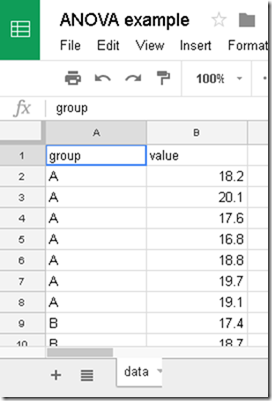##### 執行R腳本 / Excute R script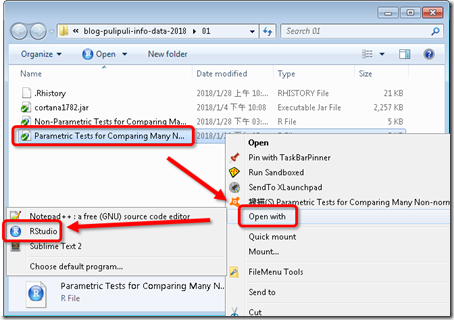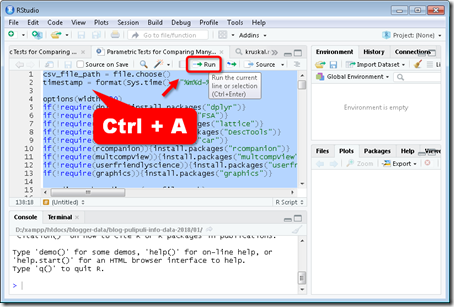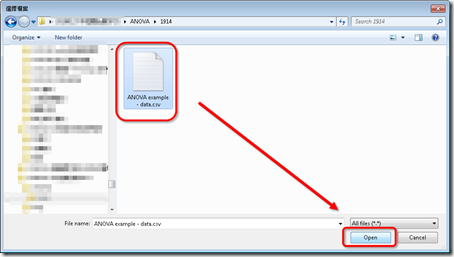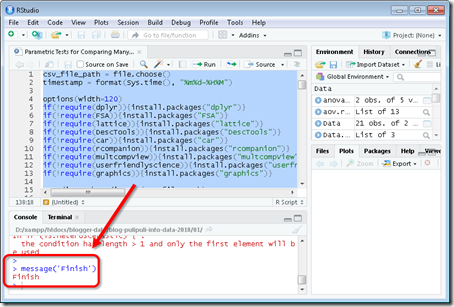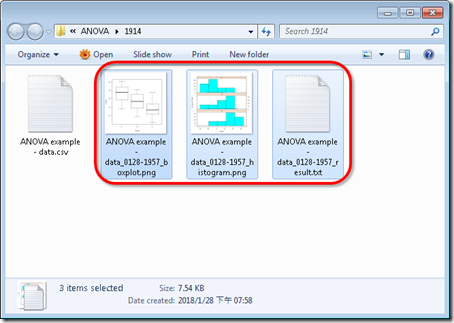• 直方圖(histogram)：檔案名稱例子為ANOVA example - data_0128-1957_histogram.png
• 箱型圖(boxplot)：檔案名稱例子為ANOVA example - data_0128-1957_boxplot.png
• 檢定結果：ANOVA example - data_0128-1957_result.txt

###### 檔案名稱 / File
``````### File

C:\data\ANOVA example - data.csv``````

###### 敘述統計 / Descriptive statistics
``````### Descriptive statistics
group n     mean       sd  min   Q1 median    Q3  max
1     A 7 18.61429 1.165373 16.8 17.9   18.8 19.40 20.1
2     B 7 17.65714 1.187234 15.9 16.9   17.7 18.55 19.1
3     C 7 16.84286 1.180194 15.2 16.2   16.7 17.40 18.8``````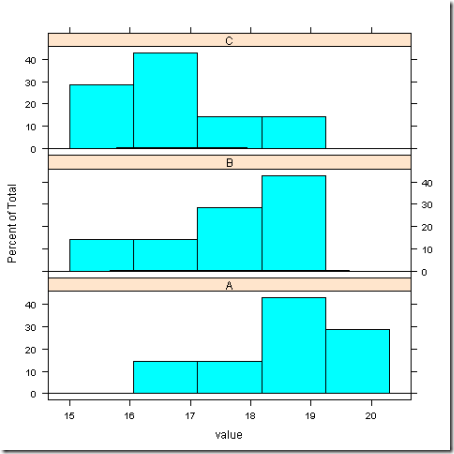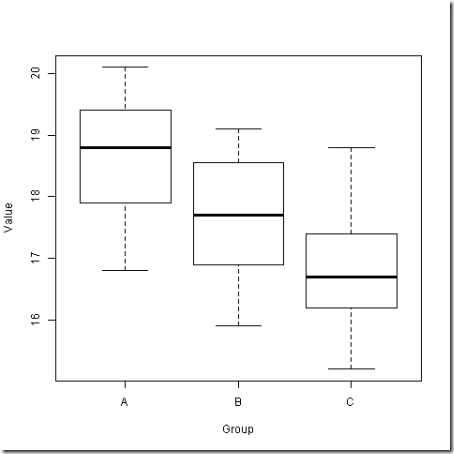###### 常態性檢定 / Normality test
``````### Normality test

Group: A, Shapiro-Wilk normality test W = 0.9756555 p-value = 0.9359176
Group: B, Shapiro-Wilk normality test W = 0.9483481 p-value = 0.7146411
Group: C, Shapiro-Wilk normality test W = 0.9878776 p-value = 0.9886119``````

###### 變異數同質性檢定 / Test for Homogeneity of Variance
``````### Test for Homogeneity of Variance

Levene's Test for Homogeneity of Variance (center = mean)       Df F value Pr(>F)
group  2  0.0162  0.984       18

Data are homoscedastic. Excute ANOVA.``````

###### 單因子變異數分析 / ANOVA
``````### ANOVA for equal variances

Analysis of Variance Table

Response: value           Df Sum Sq Mean Sq F value  Pr(>F)
group      2 11.007  5.5033  3.9683 0.03735 *
Residuals 18 24.963  1.3868
---
Signif. codes:  0 ‘***’ 0.001 ‘**’ 0.01 ‘*’ 0.05 ‘.’ 0.1 ‘ ’ 1

Eta squared: 0.3059998``````

ANOVA檢定統計量為F值(F value)，此例的F值為3.9683，顯著性p值要看Pr(>F)，本例子中F值的顯著性p值為0.03735，低於顯著水準0.05，表示三組資料的平均數有顯著的差異。

• 0.059 > eta squared >= 0.01 低度關聯強度
• 0.138 > eta squared >= 0.059 中度關聯強度
• eta squared >= 0.138 高度關聯強度

###### Scheffe法事後多重比較檢定 / Scheffe Post Hot test
``````### Post Hoc Tests
Posthoc multiple comparisons of means : Scheffe Test      95% family-wise confidence level

\$group           diff    lwr.ci      upr.ci   pval
B-A -0.9571429 -2.635501  0.72121521 0.3370
C-A -1.7714286 -3.449787 -0.09307051 0.0376 *
C-B -0.8142857 -2.492644  0.86407235 0.4493

---
Signif. codes:  0 '***' 0.001 '**' 0.01 '*' 0.05 '.' 0.1 ' ' 1``````

#### 實作：多組常態分佈異質性資料之中位數差異檢定 / Practice: Test for difference in means among many heteroscedastic groups

##### 異質性的資料 / Heteroscedastic data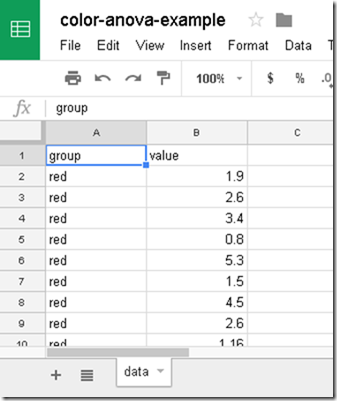##### 執行R腳本 / Excute R script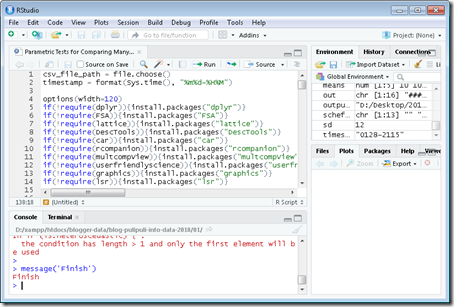• 直方圖：color-anova-example - data_0128-2115_histogram.png
• 箱型圖：color-anova-example - data_0128-2115_boxplot.png
• 檢定結果：color-anova-example - data_0128-2115_result.txt

##### 檢定結果 / Result

###### 檔案名稱 / File
``````### File

C:\data\color-anova-example - data.csv``````

###### 敘述統計 / Descriptive statistics
``````### Descriptive statistics
group  n      mean       sd  min    Q1 median    Q3  max
1   red 24  2.491667 1.546350 0.80 1.405    2.0  3.40  6.7
2 green 24  8.530417 6.977992 1.00 4.000    6.4  9.85 27.2
3  blue 24 10.632083 5.976309 5.27 6.600    8.0 11.75 25.6``````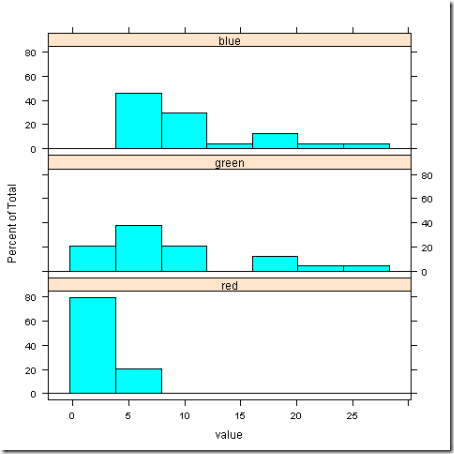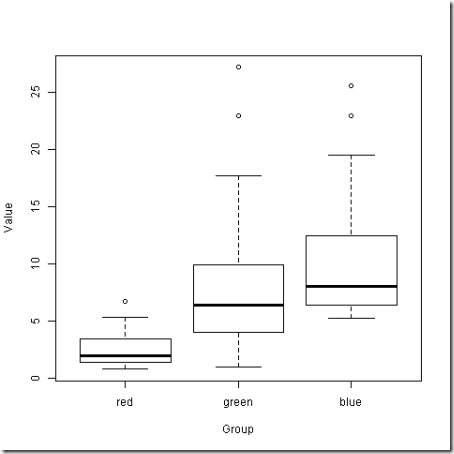###### 常態性檢定 / Normality test
``````### Normality test

Group: red, Shapiro-Wilk normality test W = 0.865417 p-value = 0.004283695
Group: green, Shapiro-Wilk normality test W = 0.8609989 p-value = 0.003522477
Group: blue, Shapiro-Wilk normality test W = 0.7905905 p-value = 0.0002075442``````

###### 變異數同質性檢定 / Test for Homogeneity of Variance
``````### Test for Homogeneity of Variance

Levene's Test for Homogeneity of Variance (center = mean)       Df F value    Pr(>F)
group  2  9.9731 0.0001568 ***       69
---
Signif. codes:  0 ‘***’ 0.001 ‘**’ 0.01 ‘*’ 0.05 ‘.’ 0.1 ‘ ’ 1

Data are heteroscedastic. Excute Welch's anova.``````

###### Welch's anova
``````### Welch’s anova for unequal variances
One-way analysis of means (not assuming equal variances)

data:  value and group
F = 27.526, num df = 2.000, denom df = 33.916, p-value = 7.898e-08``````

###### Games-Howell事後多重比較 / Games-Howell Post-Hoc Test
``````### Games-Howell Post-Hoc Test

### Oneway Anova for y=value and x=group (groups: red, green, blue)

Omega squared: 95% CI = [.12; .44], point estimate = .28
Eta Squared: 95% CI = [.14; .42], point estimate = .3
SS Df    MS     F     p
Between groups (error + effect)  857.2  2 428.6 14.81 <.001
Within groups (error only)      1996.4 69 28.93

### Post hoc test: games-howell
diff ci.lo ci.hi    t    df     p
green-red  6.04  2.41  9.67 4.14 25.25  .001
blue-red   8.14  5.01 11.27 6.46 26.07 <.001
blue-green 2.10 -2.44  6.65 1.12 44.94  .507``````

Eta Squared的點估計值為0.3，表示高度關聯強度。然後我們看「### Post hoc test: games-howell」以下的部分，可以看到「red」、「green」、「blue」三組的比較結果。

#### 結語 / Conclusion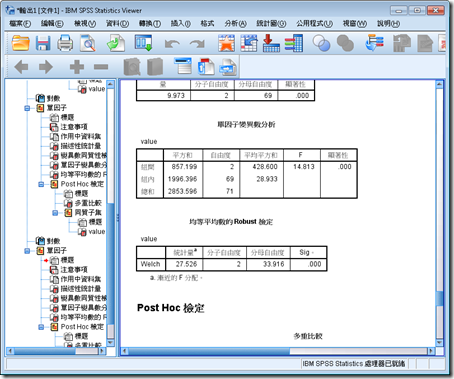#### 總共8 則留言, (我要發問)

1.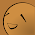如果要用RScript執行的話，那就要多加入一個指令才能安裝套件：
options(repos = "https://cran.rstudio.com")

來源：https://stackoverflow.com/a/45116122/6645399

2.RStudio預設載入了很多常用的函數，這些函數大多都在methods套件裡面
請使用以下語法來載入他們：
if(!require(methods)){install.packages("methods")}

methods套件的說明
https://www.rdocumentation.org/packages/methods/versions/3.4.3

3.R語言裡面不能在雙引號"裡面使用單引號'，例如以下是錯誤的
cat("\n### Welch’s anova for unequal variances", out, file=output_file_path, sep="\n", append=TRUE)

但是可以在單引號'裡面使用脫逸的單引號\' ，以下是正確的
cat('\n### Welch\'s anova for unequal variances', out, file=output_file_path, sep="\n", append=TRUE)

實在是常常卡在 R的眉眉角角orz

4.1.有沒有辦法進階成同時分析多組變數呢?
結果呈現為value1, value2.....
圖片的話改存為pdf?

2.To 陳昊，

我看不太懂你的意思...
是批次做多組比較的意思嗎？
例如我有A-B-C要比較，還有 甲-乙-丙 要比較的意思嗎？
這個不能分別執行兩次即可...?

如果會寫R的話，也可以用程式去抓資料夾裡面的資料進行分析

另外圖片存成PDF的部分
https://www.stat.berkeley.edu/~paciorek/computingTips/Saving_graphics_as_pdf_file.html
看起來可以用這個語法來輸出成PDF
pdf(file.pdf,width=6,height=4,paper='special')

這個就請自行研究與修改吧
開放原始碼就是可以自己動手做

5.這個腳本實在太好用了，非常的感謝!!
整個脈絡跟教學也寫得很清楚，
讓我這幾天花了不少時間泡在貴Blog
很有收穫，再次感謝!!

1.To 黑凱文，

不客氣，有問題也可以提出，我能回答就幫忙回答。